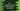# C++ program to store student details using class# Introduction :

In this C++ tutorial, we will learn how to read the details of students and how to print it using a Class. We will use one class to hold the name and marks of each student and two member functions to read, print these details.

I am explaining the steps at the end of this article. You can go through the steps to learn how the program works.

## C++ program :

``````#include <iostream>
#include <string>
using namespace std;

// 1
class Student
{
private:
string name;
int marks;

public:
void getDetails();
void setDetails();
};

// 2
void Student::setDetails()
{
cout << "Enter the name : " << endl;
cin >> name;
cout << "Enter total marks : " << endl;
cin >> marks;
}

// 3
void Student::getDetails()
{
cout << "Name : " << name << " ,marks : " << marks << endl;
}

int main(int argc, char const *argv[])
{
// 4
int count;
cout << "Enter the count of students : ";
cin >> count;

// 5
if (count > 0)
{
// 6
Student studentArray[count];
for (int i = 0; i < count; i++)
{
cout << "For student " << i + 1 << " :" << endl;
studentArray[i].setDetails();
}

// 7
cout << "\nYou have entered : " << endl;
for (int i = 0; i < count; i++)
{
studentArray[i].getDetails();
}
}
else
{
cout << "Please enter a valid number." << endl;
}
return 0;
}``````

### Explanation :

The commented numbers in the above program denote the step numbers below :

1. We have one Student class with two private variables and two public methods. Private variables name and marks are used to store the name and marks of a student and getDetails, setDetails are used to get the details and assign values to the private variables.
2. This is a member function to set values to the variable’s name and marks. If you call this function, it will ask the user to enter the name and marks. Using cin, we are storing these values to variables name and marks.
3. This member function is used to print the details. It prints the name and marks for that class object.
4. The main function is the start point. Ask the user to enter the total number of students. Read this value and store it in count.
5. Check if the entered value is greater than 0 or not. If not, print one message to enter a valid number.
6. If the entered value is greater than 0, create one array of Student to hold the students names and marks. Using one for loop, we are assigning the values to these variables. Just call the setDetails function to a Student object, and it will set the name and total marks.
7. Use one more for loop to print the user entered values. We are using the getDetails method to print the name and marks.

### Sample output :

#### Example 1 :

``````Enter the count of students : 4
For student 1 :
Enter the name :
Alex
Enter total marks :
88
For student 2 :
Enter the name :
Bob
Enter total marks :
77
For student 3 :
Enter the name :
Charlie
Enter total marks :
66
For student 4 :
Enter the name :
Dan
Enter total marks :
59

You have entered :
Name : Alex ,marks : 88
Name : Bob ,marks : 77
Name : Charlie ,marks : 66
Name : Dan ,marks : 59``````

#### Example 2 :

``````Enter the count of students : -3